Algebra 2 4-7 Complete Lesson: The Quadratic Formula
starstarstarstarstarstarstarstarstarstar
5 (1 rating)
by Matthew Richardson
| 26 Questions
Note from the author:
A complete formative lesson with embedded slideshow, mini lecture screencasts, checks for understanding, practice items, mixed review, and reflection. I create these assignments to supplement each lesson of Pearson's Common Core Edition Algebra 1, Algebra 2, and Geometry courses. See also mathquest.net and twitter.com/mathquestEDU.The outlined content above was added from outside of Formative.
1
10 pts
Solve It! On this happy face, what quadratic functions have graphs that meet the following conditions? Consider only the domain 2 ≤ x ≤ 6.

◆ crosses the x-axis twice
◆ touches the x-axis only once
◆ misses the x axis completely

• crosses the x-axis twice
• touches the x-axis only once
• misses the x axis completely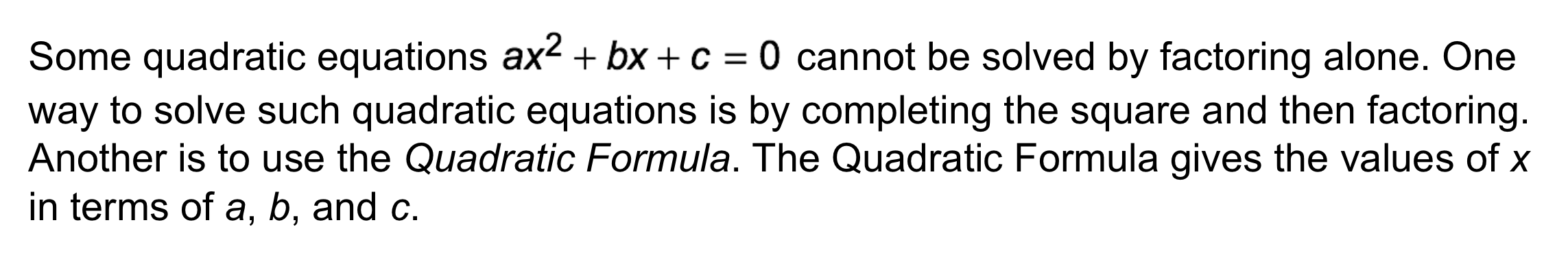2
2
10 pts
Problem 1 Got It?
A
B
C
D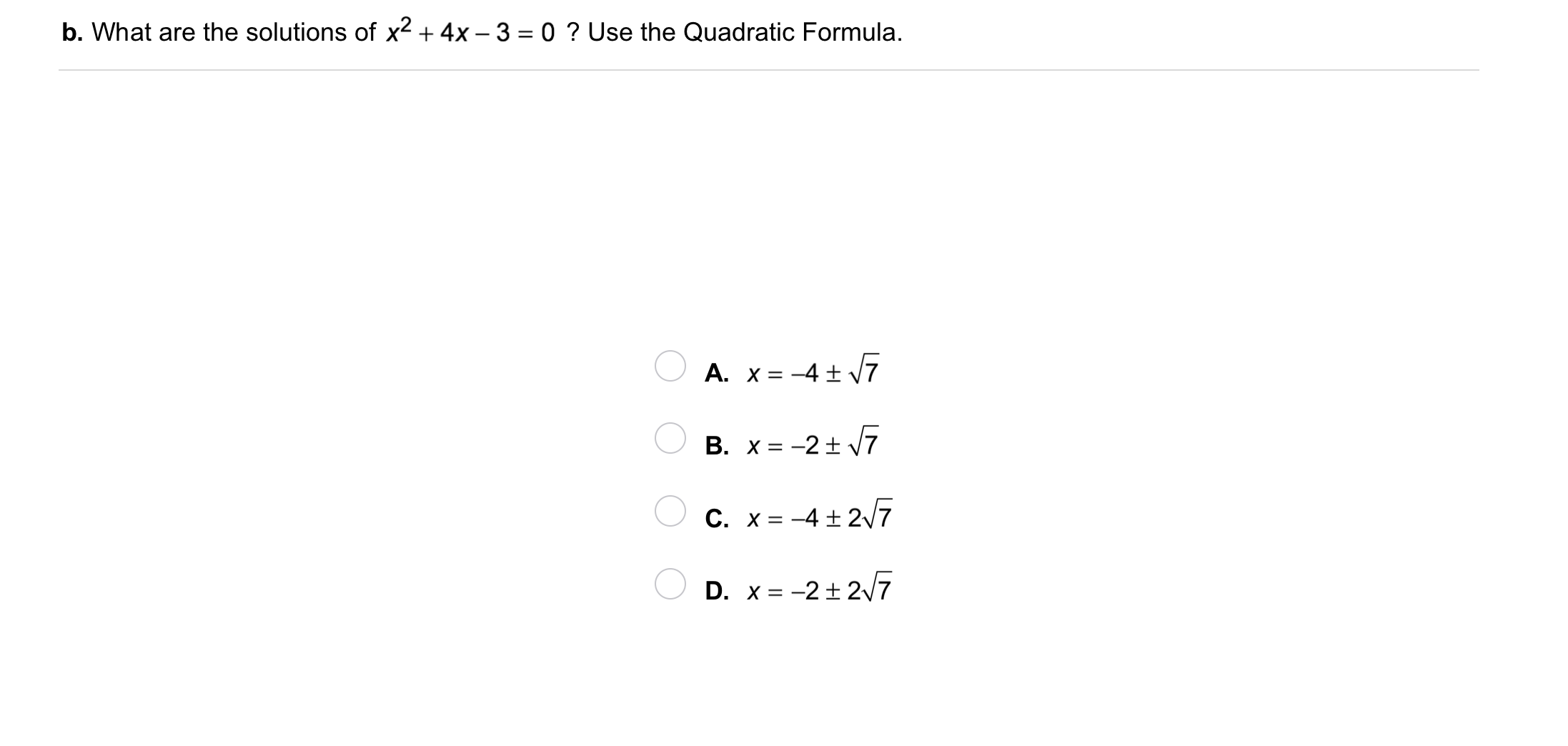3
3
10 pts
Problem 1 Got It?
A
B
C
D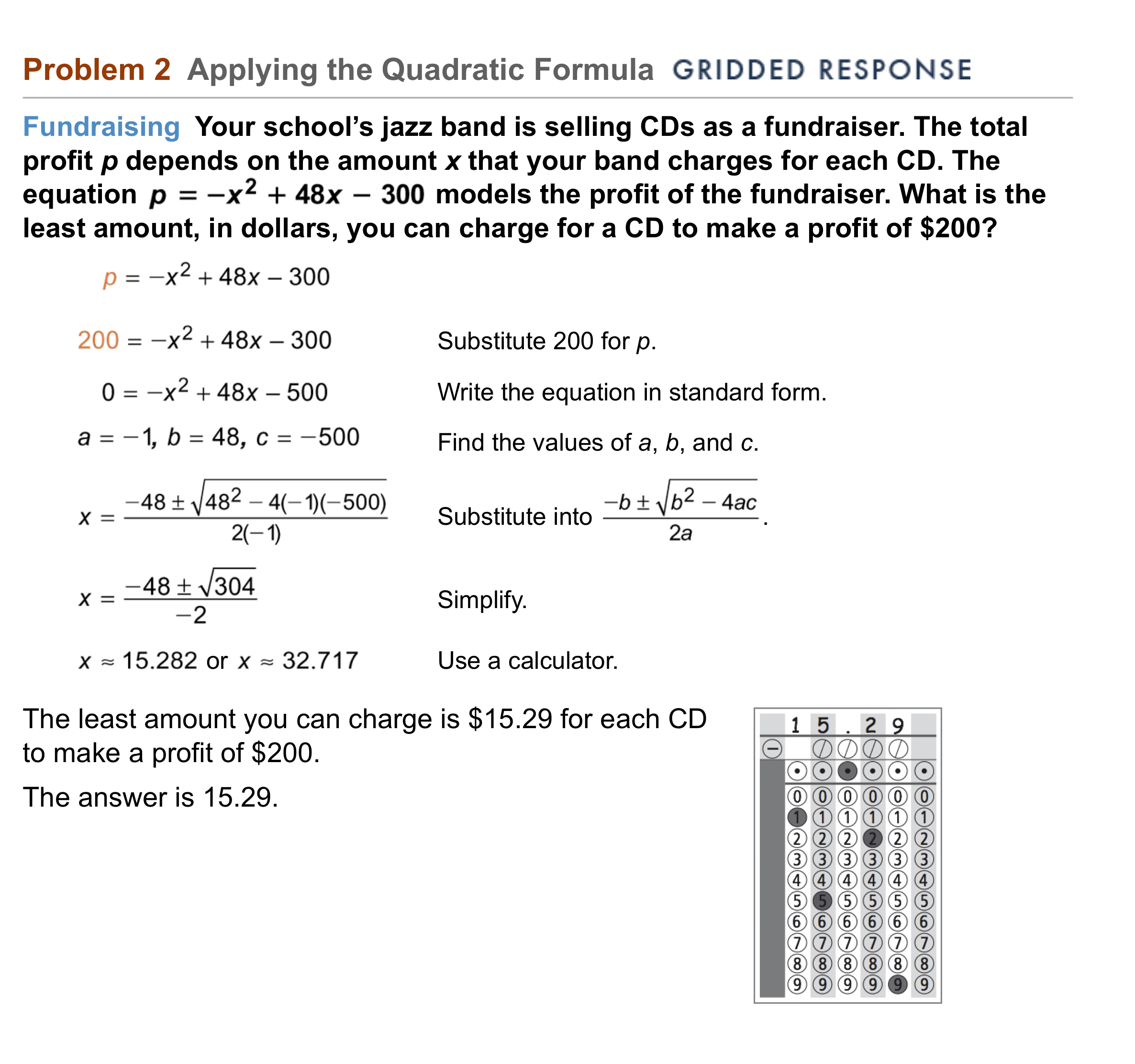4
5
4
10 pts
Problem 2 Got It? In Problem 2, what is the least amount you can charge for a CD to make a \$100 profit?
\$15.29
\$10.74
\$13.20
\$12.29
5
10 pts
Problem 2 Got It? Reasoning: Would a negative profit make sense in Problem 2? Explain.
No; Only positive quantities can represent profit.
Yes; A negative profit means that more money was spent than earned.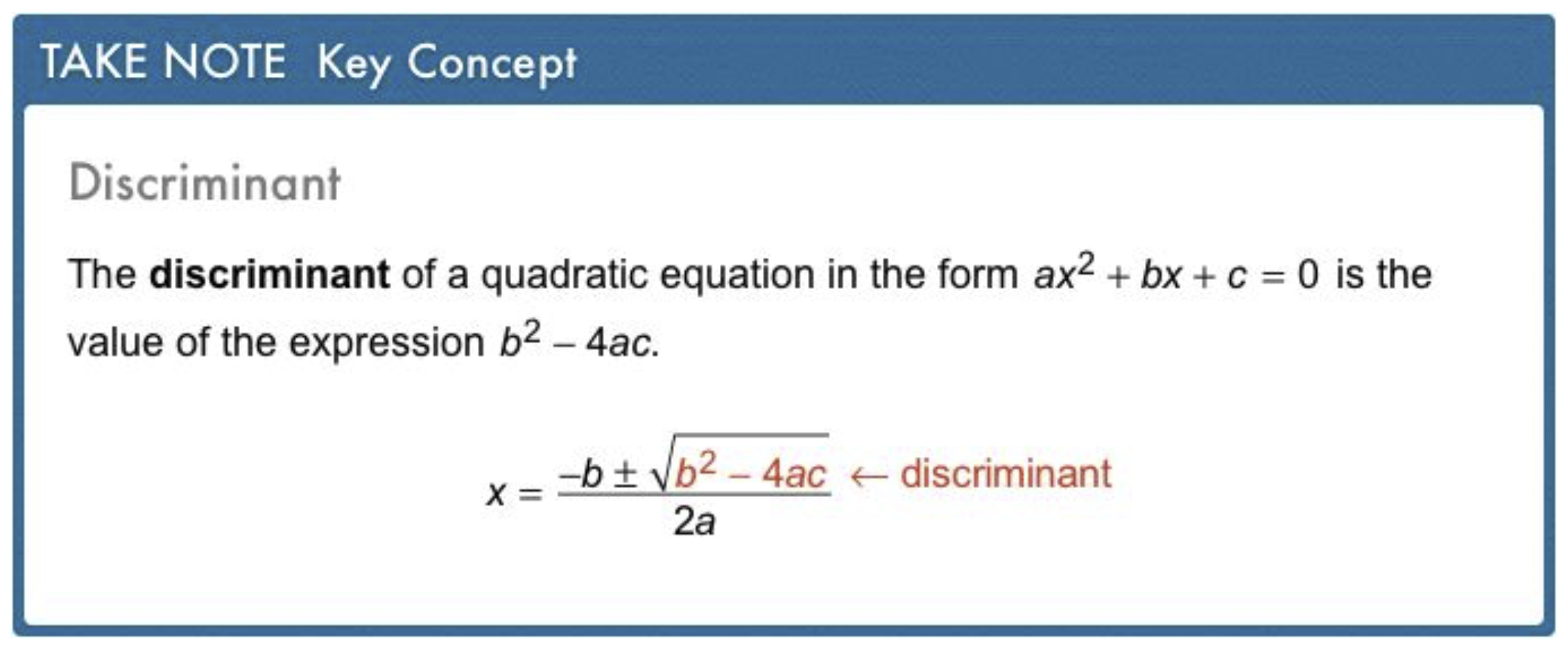6
6
10 pts
Problem 3 Got It?
A
B
C
D7
7
10 pts
Problem 3 Got It?
A
B
C
D8
8
10 pts
Problem 4 Got It? Reasoning: Will the golf ball in Problem 4 ever reach a height of 110 ft? Use the discriminant to explain your answer.
No; The discriminant would still be negative meaning the related equation has no real solutions.
Yes; The discriminant would be positive so the related equation has 2 real solutions.9
9
10 pts
A
B
C
D10
10
10 pts
A
B
C
D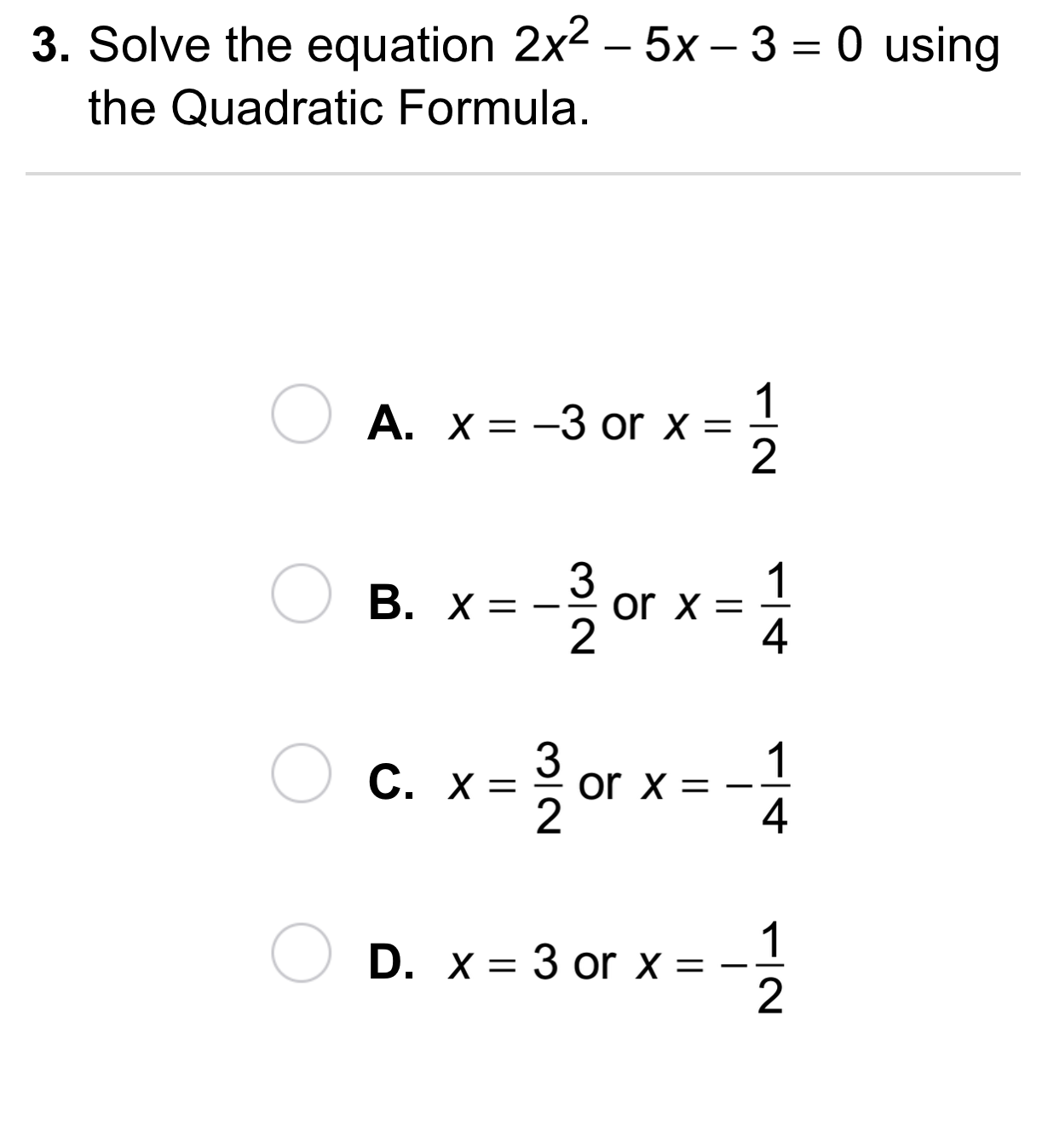11
11
10 pts
A
B
C
D12
12
10 pts
A
B
C
D13
13
10 pts
A
B
C
D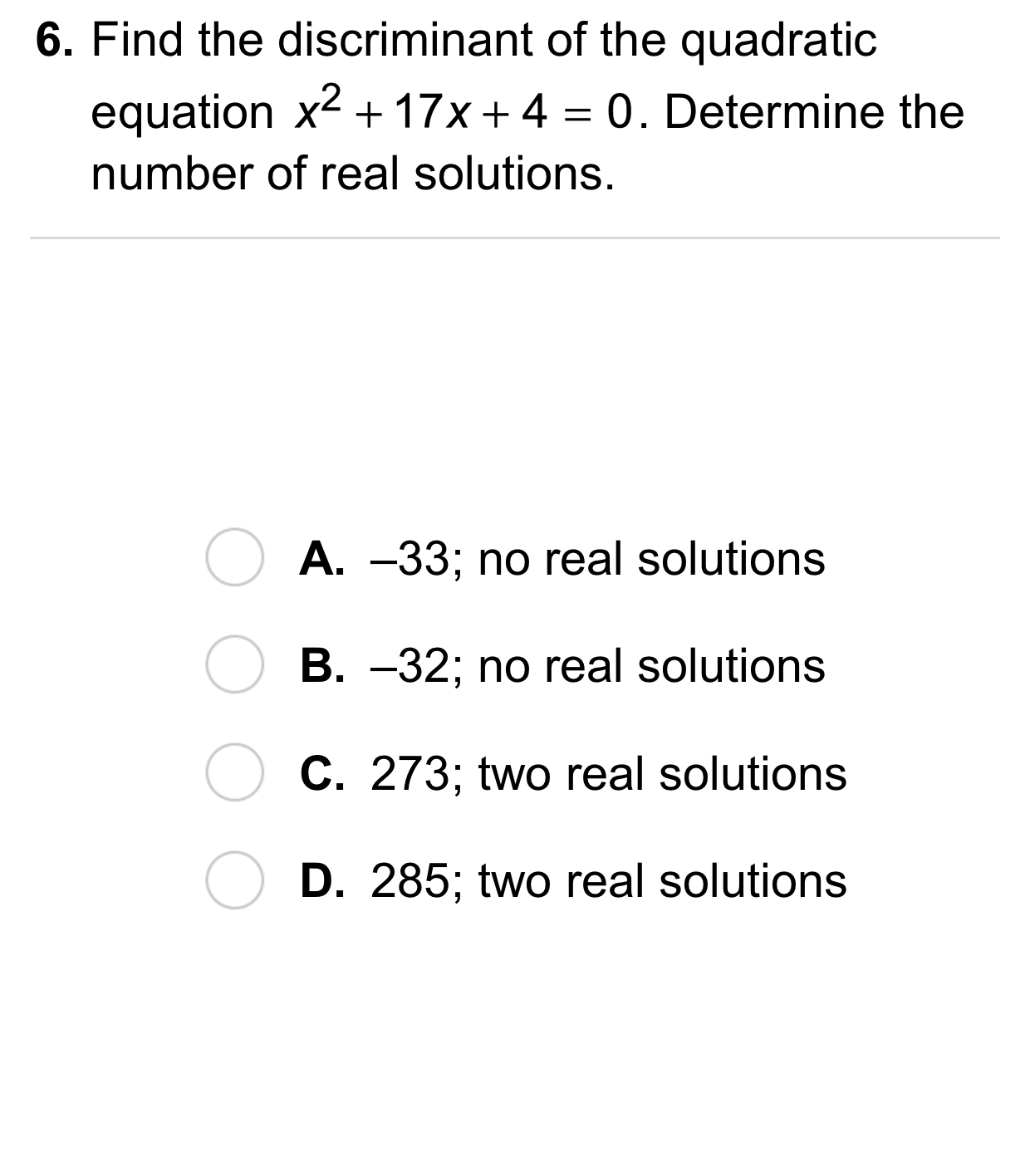14
14
10 pts
A
B
C
D15
15
10 pts
A
B
C
D16
10 pts
Reasoning: For what value(s) of k does the equation below have one real solution? two real solutions?

• k = 6
• k > 6
• k < 6
• The equation has one real solution for the following value(s) of k.
• The equation has two real solutions for the following value(s) of k.
17
10 pts
Error Analysis: Your friend concluded that because two discriminants are equal, the solutions to the two equations are the same. Explain your friend's error. Give an example of two quadratic equations that disprove this conclusion.
18
10 pts
Reasoning: If one quadratic equation has a positive discriminant, and another quadratic equation has a discriminant equal to 0, can the two quadratic equations share a solution? Explain why or why not. If so, give two quadratic equations that meet this criterion.19
20 pts
Review Lesson 4-6: Solve the equation by completing the square. Show your work on the canvas.

20
10 pts
Review Lesson 1-3: Simplify by combining like terms. Match each expression on the left with its simplified form on the right.

• 9 + 4x + 4y
• 9 + 3y
• 4- 8x - 2y
21
10 pts
Review Radical Expressions: Match each radical expression on the left with its simplified form on the right.22
10 pts
Vocabulary Review: Match each formula with its description.

• area of a square
• area of a circle
• circumference of a circle
• perimeter of a rectangle23
10 pts
Use Your Vocabulary: Identify the discriminant of the equation.

24
10 pts
Use Your Vocabulary: Identify the discriminant of the equation.

25
100 pts
Notes: Take a clear picture or screenshot of your Cornell notes for this lesson. Upload it to the canvas. Zoom and pan as needed.

For a refresher on the Cornell note-taking system, click here.
26
10 pts
Reflection: Math Success
Add to my formatives list

Formative uses cookies to allow us to better understand how the site is used. By continuing to use this site, you consent to the Terms of Service and Privacy Policy.